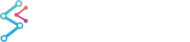﻿ Axis Types in SciChart3D | JavaScript Chart DocumentationSciChart.js JavaScript 3D Charts API > Axis3D APIs > Axis Types in SciChart3D
Axis Types in SciChart3D

SciChart 3D Android features several axis types. All inherit from AxisBase3D. The Axis are the logical representation of the XZ, ZY, YX planes in the Axis Cube.

Axes are required to measure the RenderableSeries, for instance, an axis is responsible for the transformation between data-values (provided by your code) and world coordinates (X,Y,Z values in 3D Space).

NOTE: It is necessary to declare XAxisYAxis and ZAxis in code before the 3D chart will draw.

Axis types available in SciChart3D are listed below.

## NumericAxis3D

The NumericAxis3D is a Value-Axis and is suitable when the data on that axis is numeric (e.g. double, it, long, float, short). It is not suitable for when the data-type is DateTime or TimeSpan.

Declare a NumericAxis3D as follows.

NumericAxis3D
Copy Code
```final NumericAxis3D xAxis = new NumericAxis3D();

xAxis.setGrowBy(new DoubleRange(0.1, 0.1));
xAxis.setVisibleRange(new DoubleRange(1.1, 2.7));
xAxis.setAxisTitle("XAxis");
```

## DateTimeAxis3D

The DateTimeAxis3D is a Value-Axis and is suitable when the data on that axis is a DateTime.

Declare a DateTimeAxis3D as follows.

DateTimeAxis3D
Copy Code
```final DateAxis3D xAxis = new DateAxis3D();

xAxis.setGrowBy(new DoubleRange(0.1, 0.1));
xAxis.setAxisTitle("XAxis");
xAxis.setAutoRange(AutoRange.Always);
xAxis.setVisibleRange(new DateRange(dateMin, dateMax));
```

## LogarithmicNumericAxis3D

The LogarithmicNumericAxis3D is a Logarithmic Value-Axis and is suitable when the data on that axis is a Numeric value e.g. Double, Int, Long, Float.

Declare a LogarithmicNumericAxis3D as follows.

LogarithmicNumericAxis3D
Copy Code
```final LogarithmicNumericAxis3D xAxis = new LogarithmicNumericAxis3D();

xAxis.setGrowBy(new DoubleRange(0.1, 0.1));
xAxis.setAxisTitle("XAxis");
// Specifies how lables appear on the axis
xAxis.setScientificNotation(ScientificNotation.LogarithmicBase);
xAxis.setTextFormatting("#.#E+0");
// Specifies the logarithm base for the logarithmic scale of the axis
xAxis.setLogarithmicBase(10d);
xAxis.setVisibleRange(new DoubleRange(0.1d, 1000d));
```